# The hunter and the rabbit

In this post I will tell you the story of the hunter and the rabbit. To keep you interested let me say that in the story we will see a powerful (yet trivial) inequality that I learned today from Yuval Peres, which he calls a reversed second moment method (we will also review the basic second moment method).

We consider a sequential game between a hunter and a rabbit. At each time step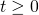the hunter (respectively the rabbit) is at a certain vertex(respectively at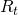) in the cycle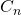(with vertices labeled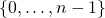). We impose the constraint thatandmust be neighbors, that is the hunter can only move one step at a time. We impose no restriction on the rabbit (he can jump around like a real rabbit). Let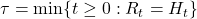be the capture time. We allow for randomized strategies for the two players.Of course the hunter wants to minimize the expected capture time while the rabbit wants to maximize it.

December 3rd clarification: the processesandare independent, in other words the hunter does not see the rabbit and vice versa (the game would be trivial otherwise!).

Theorem [Adler et al., 2003]: The hunter can always ensureand the rabbit can always ensure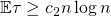.

We will now sketch the proof of this beautiful theorem following the method of [Babichenko et al. 2012]. Quite intuitively it is enough to show that there exists a strategy for the hunter that ensures for any deterministic rabbit, and respectively that there exists a strategy for the rabbit that ensures for any deterministic hunter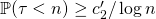(see Lemma 2.2. here for a formal proof of this).

The hunter’s strategy

Letbe independent uniform random variables on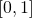. The location of the hunter at timeis. In some sense this hunter moves at a random speed determined by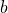and in a random direction determined by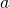. The key idea to analyze this strategy is to consider the number of collisions before time. Clearly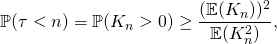where the inequality is a ‘strong’ form of the second moment method which can be proved with Cauchy-Schwarz as follows (recall that the basic second moment simply says):Now it is just a matter of computingandwhich can be done very easily, see page 9 here.

The rabbit’s strategy

The rabbit’s strategy is an order of magnitude more complicated/more beautiful. The basic intuition to design a good rabbit’s strategy is the reversed second moment method which is the following trivial inequality: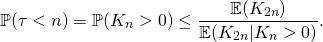Let us look into this last term in more details. Of course the rabbit’s location at a given time step should be uniformly distributed, thus the numerator will be equal to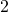(recall that here the hunter is deterministic). Thus we want to maximize the denominator, in other words once the rabbit has collided with the hunter, he should try to collide as much as possible after that! This is in my opinion a truly beautiful intuition. The way to achieve this proposed in [Babichenko et al. 2012] goes as follows. Let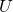be a random variable uniformly distributed inandbe a simple random walk (independent of) in. Letand for,The rabbit is then defined by(with). Using basic properties of the simple random walk in 2 dimensions one can analyze the term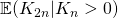in a few lines, see page 7 here. I suspect that one could come up with other rabbit strategies that achieve the same excepted capture time.

This entry was posted in Probability theory. Bookmark the permalink.

## 2 Responses to "The hunter and the rabbit"

••